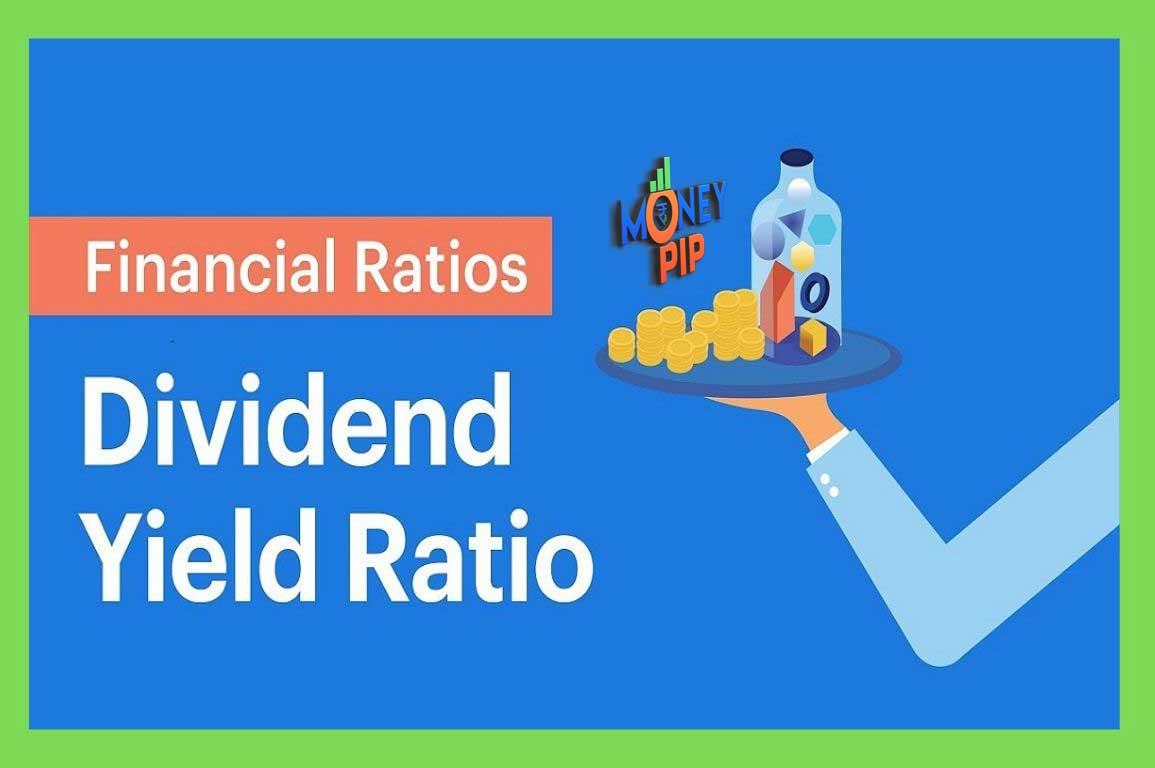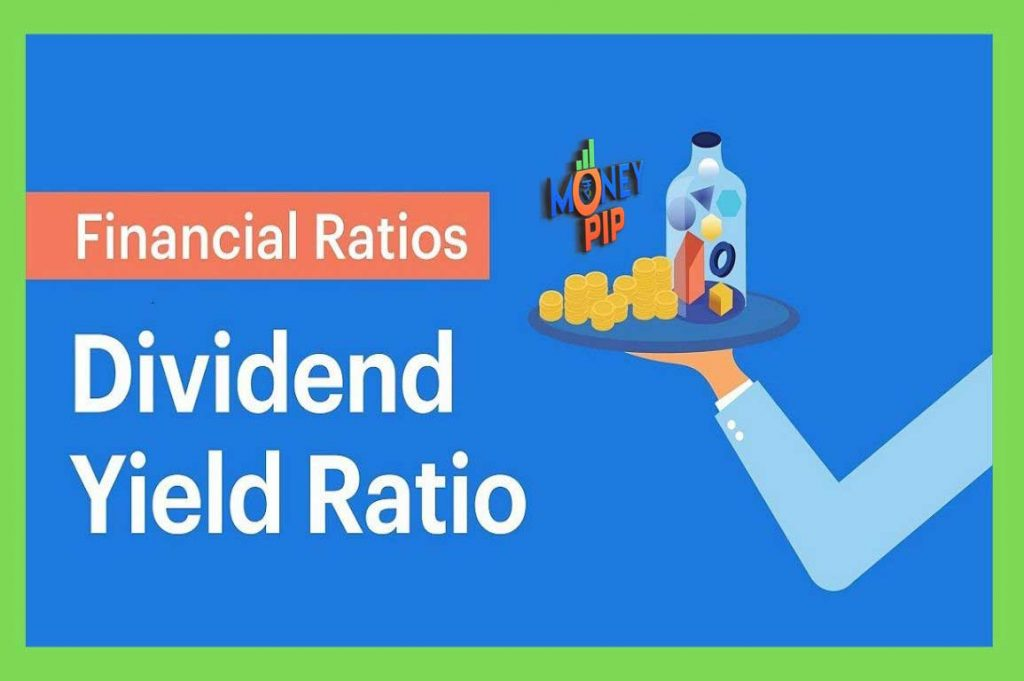Dividend Yield Ratio

Table of Contents

## Dividend Yield Ratio: Calculation, Formula, Analysis, Explanation

In this Article we will talk about dividend yield.  If you want to know about two more financial ratios, Debt to  Equity ratio and Return on Equity ratio,    you can Read our previous two article. Where we have talked about them.

## Dividend Yield Ratio Meaning

Friends, now let’s move to the third  ratio, which is Dividend Yield. It’s a valuation ratio. Dividend Yield ratio is a ratio which tells you how much dividend per share you are going to get  for the per share price of the company.

For example, if the price of the company is Rs. 200 and if you are expecting,  or the last reported dividend is Rs. 5, this means that the Dividend Yield  of the company is 2.5%. This is how you calculate  dividend yield of a company.

In different industries, in different companies, there are different yields.    It’s not necessary that high dividend yield company is always better than low dividend yield company. The base reason for this is that sometimes  companies do not pay a dividend at all and they reinvest their entire profit  to expand their business or to improve the business operation.

In this case, the company has the potential to grow a lot in the future  and give you more dividends. Your probability of getting more dividends  in the future increases here. Company didn’t give a dividend this year, but it may happen that five years down the line, it may pay more dividends.

Hence, dividend of this company  is almost zero or let’s say it paid very little dividend. So, dividend yield is less. As compared to company which, let’s say, are giving 40% of its  net profit as a dividend. If it’s price is, let’s say, Rs. 100, and  it gave a dividend of Rs. 40 per share, then its dividend yield will be 40%.

READ  How the whole Stock Market Works

From this, you may guess that company doesn’t have many business opportunities to invest its net profit. So, it is giving  more Dividends to its shareholders.Dividend Yield Ratio

### How we are Calculating  the Dividend Yield

Friends, the second important point  here is, dividend per share divided by  price per share of the company.  This is how we are calculating  the dividend yield. Here, if the price of the company,  which changes daily, if price of any company is very volatile, dividend yield will change daily.

Because, dividend, which is the numerator, Dividend per share, remains constant. But price changes daily. Hence, our  dividend yield will fluctuate daily.

For example, recently,  you might have heard in the news that, Indiabulls Housing Finance stock crashed in the last two weeks. Basically, its price was around 400 and it gave latest reported  dividend of Rs. 40/per share. So, its dividend yield was 40/400,  which was 10%.  Now, its price is around 200, after the crash, because of some reasons. And its dividend yield automatically became 20% from 10%.

So, as I have told you just now, as soon as its price reduced to half, from 400 to 200, now the denominator is 200  but dividend is still the same. because your numerator is such a figure  which always remains mostly constant  which is the last reported figure.

Here, you saw that how its dividend  shot from 10% to 20%  within a span of, let’s say, 15 days or four months. That’s what I explained to you if the price is fluctuating too much, if its a very volatile stock, you will see dividend yield changing greatly. This is the way this financial ratio, which is a valuation ratio helps you to determine how much dividend you can get from a company and how much you should expect.

READ  How to Apply Reliance Rights Issue Step-by-Step

Friends, today we talked about  dividend yield ratio. In the next we will talk about PE ratio and Free Cash Flow to Sales ratio. Make sure to follow on Google News, to get the notification as soon as our next article arrives.